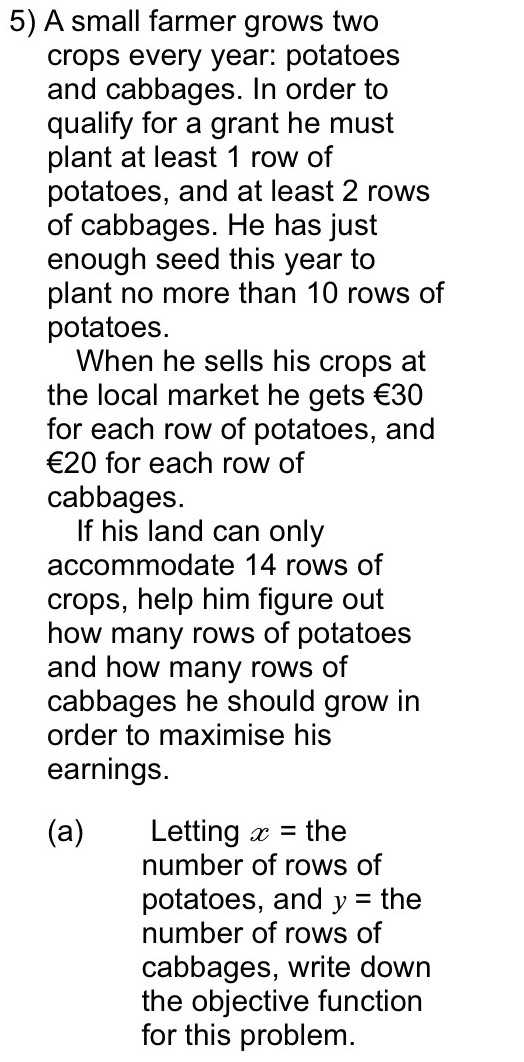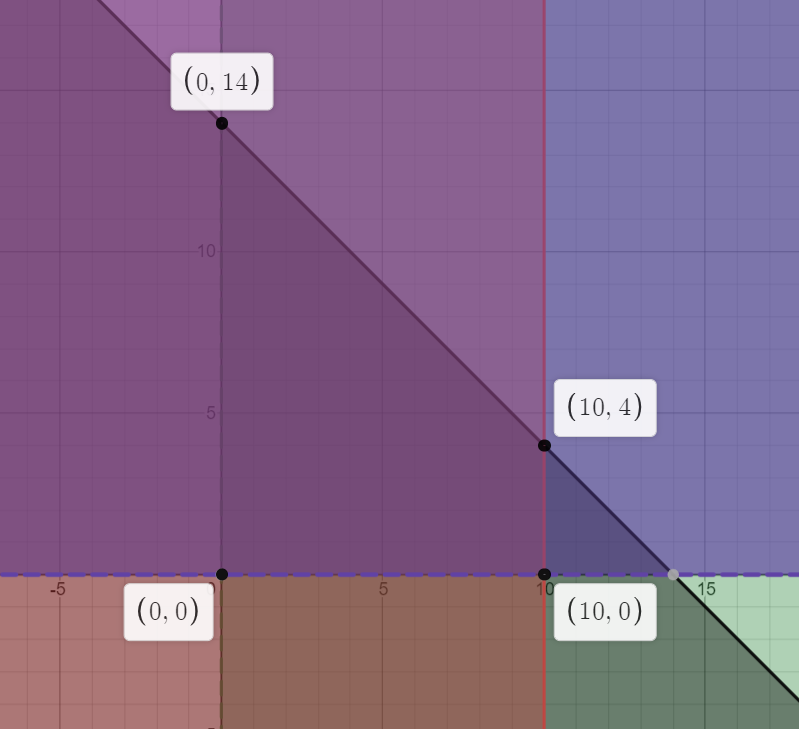### ¿Todavía tienes preguntas de matemáticas?

Pregunte a nuestros tutores expertos
Algebra
Pregunta5) A small farmer grows two crops every year: potatoes and cabbages. In order to qualify for a grant he must plant at least $$1$$ row of potatoes, and at least $$2$$ rows of cabbages. He has just enough seed this year to plant no more than $$10$$ rows of potatoes.

When he sells his crops at the local market he gets $$€ 30$$ for each row of potatoes, and $$€ 20$$ for each row of cabbages.

If his land can only accommodate $$14$$ rows of crops, help him figure out how many rows of potatoes and how many rows of cabbages he should grow in order to maximise his earnings.

(a) Letting $$x =$$ the number of rows of potatoes, and $$y =$$ the number of rows of cabbages, write down the objective function for this problem.

x > 0 , y > 0  , x + y $$\le$$14 , x $$\le$$ 10 ,  Z = 30x + 20y  ,solution x = 10 , y = 4